• python画图写文字
千次阅读
2020-12-29 08:08:02

Matplotlib使用总结图%matplotlib inline

import matplotlib.pyplot as plt

plt.rcParams['font.sans-serif']=['SimHei'] # 用来正常显示中文标签

plt.rcParams['axes.unicode_minus']=False # 用来正常显示负号

import pandas as pd

import numpy as np1

2

3

4

5

6

7

字体

import os

from matplotlib import font_manager as fm, rcParams

import matplotlib.pyplot as plt

fig, ax = plt.subplots()

fpath = os.path.join(rcParams["datapath"], "fonts/ttf/cmr10.ttf")

prop = fm.FontProperties(fname=fpath)

fname = os.path.split(fpath)

ax.set_title('This is a special font: {}'.format(fname), fontproperties=prop)

ax.set_xlabel('This is the default font')

plt.show()1

2

3

4

更多相关内容
• 今天在一个研究生创新项目申报书时涉及到一个python画图问题，对于在x轴各个区段显示自定义的字符串有些疑问，特此记录。界面如下所示：代码如下所示：import matplotlib.pyplot as pltfrom pylab import * #支持...今天在写一个研究生创新项目申报书时涉及到一个python画图问题，对于在x轴各个区段显示自定义的字符串有些疑问，特此记录。

界面如下所示：

代码如下所示：

import matplotlib.pyplot as plt

from pylab import * #支持中文

mpl.rcParams['font.sans-serif'] = ['SimHei']

"""条形图bar"""

x = ['10/Q1', '10/Q3', '11/Q1', '11/Q3', '12/Q1', '12/Q3', '13/Q1', '13/Q3', '14/Q1', '14/Q3', '15/Q1', '15/Q3', '16/Q1', '16/Q3', '17/Q1', '17/Q3']

y = [20, 35, 39, 62, 87, 114, 140, 169, 187, 211, 225, 239, 241, 247, 251, 258]

# plt.bar([1,3,5,7,9],[5,2,7,8,2],label='Example One',color='b')#plt.bar创建条形图

# plt.bar([2,4,6,8,10],[8,6,2,5,6],label='Example Two',color='g')

plt.bar(range(16), y, color = 'lightsteelblue')

plt.plot(range(16), y, marker = 'o', color = 'coral') #coral

plt.xticks(range(16), x)

plt.xlabel('年份/季度')

plt.ylabel("月活跃用户数(单位：百万)")

plt.legend()

plt.show()

以上这篇python画图系列之个性化显示x轴区段文字的实例就是小编分享给大家的全部内容了，希望能给大家一个参考，也希望大家多多支持。

展开全文• python绘图 添加文字Adding text to a plot is one of the most used features of matplotlib.pyplot and there is a function defined for this operation i.e. matplotlib.pyplot.text(). 将文本添加到绘图是...

python绘图 添加文字

Adding text to a plot is one of the most used features of matplotlib.pyplot and there is a function defined for this operation i.e. matplotlib.pyplot.text().

将文本添加到绘图是matplotlib.pyplot最常用的功能之一，并且为此操作定义了一个函数，即matplotlib.pyplot.text()

The following example shows the usage of this function.

以下示例显示了此功能的用法。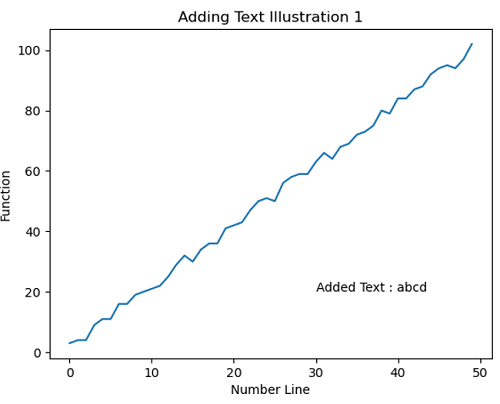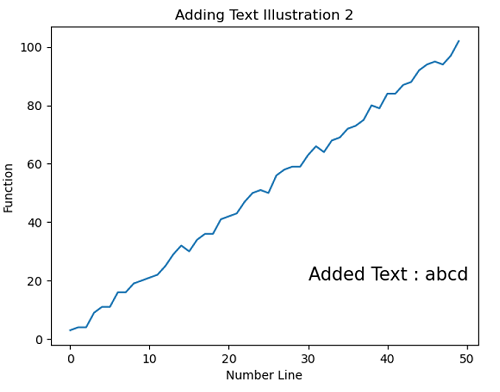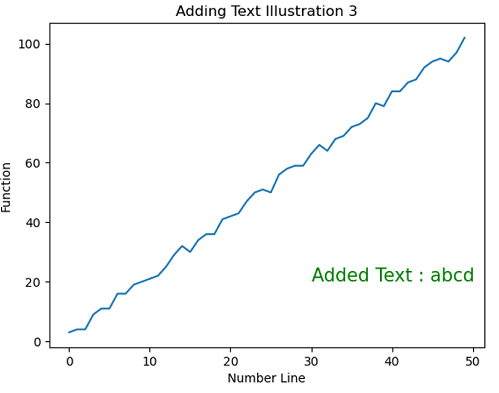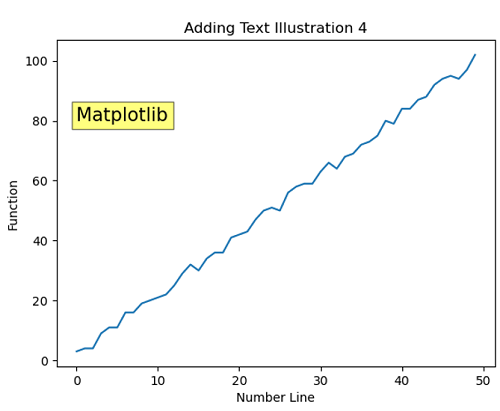Reference: https://matplotlib.org/

## 用于向绘图添加文本的Python代码 (Python code for adding text to the plot)

# Data Visualization using Python

import numpy as np
import matplotlib.pyplot as plt

x = np.arange(50)
y1 = np.arange(50)
for i in range(50):
y1[i] = 2*x[i] + np.random.randint(0,5)

# Adding Text Illustration 1
plt.figure()
plt.plot(x,y1)
plt.xlabel('Number Line')
plt.ylabel('Function')
plt.title('Adding Text Illustration 1')
plt.text(30,20,'Added Text : abcd')

# Adding Text Illustration 2
plt.figure()
plt.plot(x,y1)
plt.xlabel('Number Line')
plt.ylabel('Function')
plt.title('Adding Text Illustration 2')
plt.text(30,20,'Added Text : abcd', fontsize=15)

# Adding Text Illustration 3
plt.figure()
plt.plot(x,y1)
plt.xlabel('Number Line')
plt.ylabel('Function')
plt.title('Adding Text Illustration 3')
plt.text(30,20,'Added Text : abcd', fontsize=15, color='g')

# Adding Text Illustration 4
plt.figure()
plt.plot(x,y1)
plt.xlabel('Number Line')
plt.ylabel('Function')
plt.title('Adding Text Illustration 4')
plt.text(0,80, 'Matplotlib', bbox=dict(facecolor='yellow', alpha=0.5), fontsize=15)



Output:

输出：

Output is as figure




python绘图 添加文字

展开全文python 机器学习 matlab
• 下面添加前的效果，如图，可以看到图例部分还是默认字体，希望修改为“TimesNewRoman”在plt.legend()中添加属性prop=font。只需添加font和prop属性即可实现图2。

在 plt.legend()  中添加属性prop=font

如：

font = {'family': 'Times New Roman','size': 12,}
plt.legend(loc="lower right",fontsize = 12,prop=font)

下面添加前的效果，如图，可以看到图例部分还是默认字体，希望修改为“Times New Roman”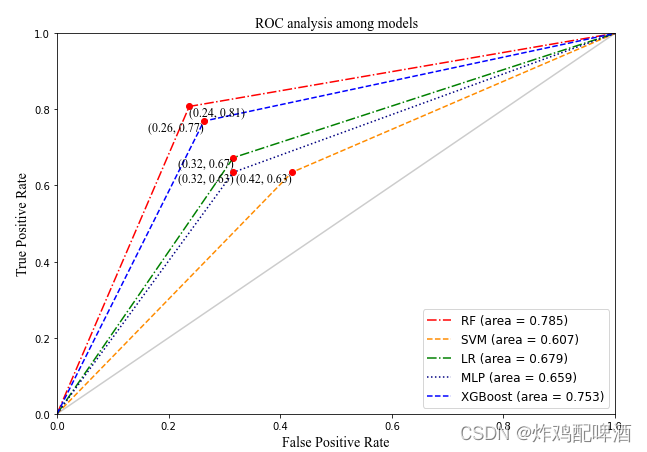只需添加font和prop属性即可实现图2

添加后的效果如图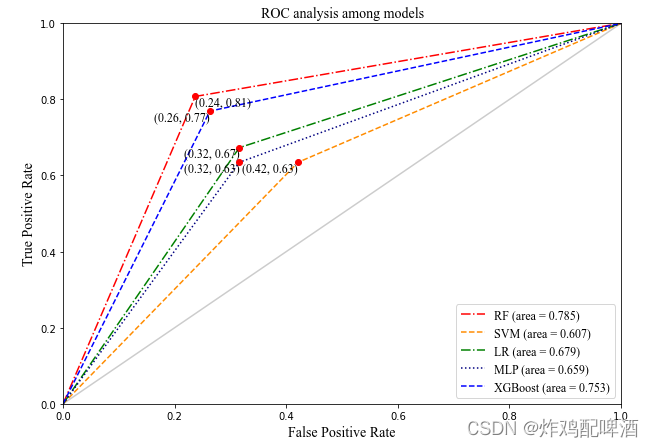展开全文python
• 在进行python 画图时 显示图片中文标题时会出现乱码。 在最前边设置这两条属性即可： plt.rcParams[‘font.sans-serif’]=[‘SimHei’] plt.rcParams[‘axes.unicode_minus’] = False 这里SimHei 表示黑体...python
• 需要有这一条命令，防止出的图中文字都变成方框 plt.legend(loc = 4,prop={"family" : "Times New Roman","size":10.5}) # 设置仿真图例说明的字体和字号 plt.xlabel(r'训练步数',fontproperties=my_font) plt....python
• 参考：Matplotlib绘图时x轴标签重叠的解决办法 - 简书 具体可以参考以上链接python
• ## python画图代码集合

千次阅读 2020-12-09 12:36:27
setp可以使用python 关键词，也可用MATLAB格式的字符串。(plt.setp(lines)) lines = plt.plot([1,2,3,4], [1,4,9,16], [1,2,3,4], [16,5,6,2]) # use keyword args plt.setp(lines, color='r', linewidth=2.0) # or...
• ## python画图系列整理

千次阅读 2022-02-05 12:51:51
python娱乐 -- 无限三角 python娱乐 -- 无限三角_Eric 的博客-CSDN博客 Python学习（一）：turtle库介绍与简单案例 Python学习（一）：turtle库介绍与简单案例_番茄哈哈的博客-CSDN博客_turtle库 python练习...python 人工智能 计算机考研
• 采用Matplotlib模块画图时，横纵坐标或者标题如果要书写希腊字母，该如何处理这一问题。 Matplotlib中支持LaTex语法，输入格式为： r’Δ\DeltaΔ’ #其中的Delta对应于希腊字母的Δ r’Δ\DeltaΔrv’ #对应于Δ...python
•python matplotlib
• ## python画图x轴文字斜着

万次阅读 多人点赞 2020-08-08 10:59:32
plt.xticks(rotation=70) # 倾斜70度
• ## Python 各种画图

万次阅读 多人点赞 2021-09-03 20:20:51
Python 画各种图所作的简单笔记，不断更新python matlab
• 今天小编就为大家分享一篇python实现在函数图像上添加文字和标注的方法，具有很好的参考价值，希望对大家有所帮助。一起跟随小编过来看看吧
• 一张字体调整好的示例图： 字体大小就是 fontsize 参数 import matplotlib.pyplot as plt # 代码中的“...”代表省略的其他参数 ax = plt.subplot(111) # 设置刻度字体大小 plt.xticks(fontsize=20) ...
• Python画图设置宋体和新罗马Times New Roman 相信很多用Python进行画图的小伙伴会有困惑，每次画出来的图都是黑体，粘贴到Word里面，和其他的文字也很不搭，但是又懒得改，主要是我一直也没找到很有效的方法，但今天...python
• python画图设置渐变色文字+代码演示发布时间：2020-05-09 14:22:36来源：亿速云阅读：501如何使用python画图设置渐变色？这个问题可能是我们日常工作经常见到的。通过这个问题，希望你能收获更多。下面是解决这个...
• 在工作中有时候会给图上添加文字，常用的是PS工具，不过我想通过代码的方式来给图片添加文字。需要使用的Python的图像库：PIL.更加详细的知识点如下：Imaga模块：用来创建，打开，保存图片文件new(path):用来创建一...
• python画图，如果用英文显示基本没有问题，但是中文可能会有乱码或者不显示的情况。 经过个人的测试，下图中“横轴”，“纵轴”字样的中文显示没有什么大问题，主要是plt.title部分和plt.plot部分的显示 plt.title...python
• 场景在用户运营中，拉新是第一步。我们产品打算先再小范围...然后就在想，能不能利用居家旅行必备的Python来处理一下？可以！所以，学习了一下：如何，简单的，在图片上，添加文字。往下拉继续看实现方式1.引入PIL...
• 懒人画图模板 https://matplotlib.org/gallery.html 配色网站 Trending color palettes - Coolors Material Design Colors - Material Palette 用Python画地图 参考资料：详解python 利用 pyecharts 画地图(热力图)...python
• ## python画图刻度向内

千次阅读 2021-10-18 15:52:11
python plt.rcParams['xtick.direction'] = 'in' # 将x周的刻度线方向设置向内 plt.rcParams['ytick.direction'] = 'in' # 将y轴的刻度方向设置向内` ![在这里插入图片描述]...python pycharm
• matplotlib官网文档：支持win7_64_bit的matplotlib库下载网址：简介：matplotlib是python最著名的绘图库，它提供了一整套和matlab相似的命令API，十分适合交互式地进行制图。而且也可以方便地将它作为绘图控件，嵌入...
• 每件事情总有两面性，当我们看到不太好的一面时，一定要想着去寻找一下好的一面。... 对于学术研究来说，当前的绘图知识，远不能支撑一个很好的研究成果的呈现，因此，对python画图的学习，还要继续深入探索和学习~
• 然后将它放置到字体路径下: python3.7/site-packages/matplotlib/mpl-data/fonts/ttf （大概是长这样） 如果你不知道路径在哪儿，在命令行里输入 python -c "import matplotlib; print(matplotlib.matplotlib_fname...python 编程语言
• 画图的时候经常需要添加上标或者下标，最常用的方法就是使用latex进行，之前就直接百度了代码复制了，但是发现有时候latex打印出来的字体和我原本的字体并不一样，于是就去找了一下原因。 首先当我们进行打印上标...python...